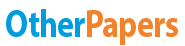# The Sensitivity of Bond Prices Week 3 Assignment

Essay by   •  June 29, 2019  •  Essay  •  703 Words (3 Pages)  •  433 Views

## Essay Preview: The Sensitivity of Bond Prices Week 3 Assignment

Report this essay
Page 1 of 3

A.  For a given coupon rate, the sensitivity of bond prices to changes in interest rates increases at an increasing rate as maturity increases. FALSE

The longer the maturity of a bond, the more sensitive is its price to a change in interest rates. The price sensitivity of any bond increases with its maturity, but the increase occurs at a decreasing rate, not an increasing rate!

B. For a given absolute change in interest rates from the same base level, the proportionate increase in bond prices when rates fall is larger than the proportionate decrease in bond prices when rates rise. – True

C.  For identical coupon rates and a given absolute change in interest rates from the same base level, long-term bonds change proportionately more in price than short-term bonds. TRUE. They have greater duration and therefore greater rate sensitivity.

D. For identical maturities and a given absolute change in interest rates from the same base level, low-coupon bonds change proportionately less in price than high-coupon bonds.

False. Duration is greater for lower coupon bonds and their interest rates are greater interest rate sensitivity as well.

[pic 1]

3. Consider the dividend discount model, the capital asset pricing model, the arbitrage pricing model and the firm valuation model.  If these accurately portray the intrinsic values of stock prices and stock returns, then what is the corresponding fundamental relationship between stock prices and interest rates?  Explain your answers.

Dividend discount model: The dividend discount model is a way of valuing a company based on the theory that a stock is equal to the discounted sum of all of its future dividend payments. The dividend discount model is used to predict the price of stocks based on the net present value of future dividends.

The relation between price of stock and annual dividends and interest rates is :

P0 = [pic 2][pic 3]

The dividend discount model assumes that the first dividend is received one year after the stock is bought and the dividends are paid regularly.

Stock prices and interest rates are negatively corelated. If the interest rates increase the value of the equity is reduced as indicated by the dividend discount model. In this case, investors might consider investing in fixed income securities.

Capital asset pricing model

...

...

Download as:   txt (4.3 Kb)   pdf (270.8 Kb)   docx (571.1 Kb)
Continue for 2 more pages »
Only available on OtherPapers.com
Citation Generator

(2019, 06). The Sensitivity of Bond Prices Week 3 Assignment. OtherPapers.com. Retrieved 06, 2019, from https://www.otherpapers.com/essay/The-Sensitivity-of-Bond-Prices-Week-3-Assignment/65990.html

"The Sensitivity of Bond Prices Week 3 Assignment" OtherPapers.com. 06 2019. 2019. 06 2019 <https://www.otherpapers.com/essay/The-Sensitivity-of-Bond-Prices-Week-3-Assignment/65990.html>.

"The Sensitivity of Bond Prices Week 3 Assignment." OtherPapers.com. OtherPapers.com, 06 2019. Web. 06 2019. <https://www.otherpapers.com/essay/The-Sensitivity-of-Bond-Prices-Week-3-Assignment/65990.html>.

"The Sensitivity of Bond Prices Week 3 Assignment." OtherPapers.com. 06, 2019. Accessed 06, 2019. https://www.otherpapers.com/essay/The-Sensitivity-of-Bond-Prices-Week-3-Assignment/65990.html.